Continuous Time LTI Systems

# Continuous Time LTI Systems - Notes | Study Signals and Systems - Electrical Engineering (EE)

 1 Crore+ students have signed up on EduRev. Have you?

Continuous Time LTI Systems

In this section our goal is to derive the response of a LTI system for any arbitrary continuous input x(t). In complete analogy with the discussion on Discrete time analysis we begin by expressing x(t) in terms of impulses. In discrete time we represented a signal in terms of scaled and shifted unit impulses. In continuous time, however the unit impulse function is not an ordinary function (i.e. it is not defined at all points and we prefer to call the unit impulse function a "mathematical object"), it is a generalized function ( it is defined by its effect on other signals) .

Recall the previous discussion on the development of the unit impulse function. It can be regarded as the idealization of a pulse of width and Δ height 1/ Δ.

One can arrive at an expression for an arbitrary input, say x(t) by scaling the height of the rectangular impulse by a factor such that it's value at t coincides with the value of x(t) at the mid-point of the width of the rectangular impulse. The entire function is hence divided into such rectangular impulses which give a close approximation to the actual function depending upon how small the interval is taken to be. For example let x(t) be a signal. It can be approximated as :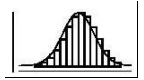The given input x(t) is approximated with such narrow rectangular pulses, each scaled to the appropriate value of x(t) at the corresponding (which lies at the midpoint of the base of width Δ . This is called the staircase approximation of x(t). In the limit as the pulse-width ( Δ ) approaches zero, the rectangular pulse becomes finer in width and the function x(t)can be represented in terms of impulses by the following expression,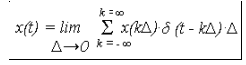This summation is an approximation. As  Δ approaches zero, the approximation increases in accuracy and when delta becomes infinitesimally small, this error becomes zero and the above summation is converted into the following integral expression.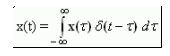For example, take x(t) = u(t)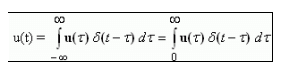since u(t) = 0 for t < 0 and u(t) = 1 for t > 0. In complete analogy with the development on sampling property of discrete unit impulse we have,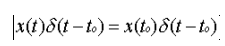This is known as Sifting Property of the continuous time impulse. Note that the unit impulse puts unit area into zero width.

The Convolution Integral

We now want to find the response for an arbitrary continuous time signal as the superposition of scaled and shifted pulses just as we did for discrete time signal. For a continuous LSI system, let h(t) be the response to the unit impulse signal. Then,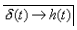by shift invariance,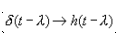by homogeneity,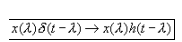by additivity, ( Note : We can perform additivity on infinite terms only if the sum/integral converges. )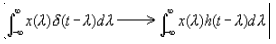This is known as the continuous time convolution of x(t) and h(t). This gives the system response y(t) to the input x(t) in terms of unit impulse response h(t). The convolution of two signals h(t) and x(t) will be represented symbolically as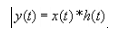To explain this graphically,

Consider the following input which (as explained above) can be considered to be an approximation of a series of rectangular impulses. And it can be represented using the convolution sum as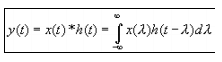Hence, by merely knowing the impulse response one can predict the response of the signal x(t) by using the given formula for convolution.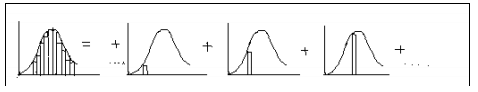RC System

Consider a RC system consisting of a resistor and a capacitor. We have to find out what the response of this system is to the unit impulse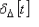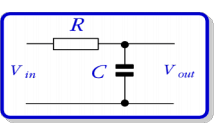First let us understand the response toIf the input is the unit step function u(t) then the output of the system will be .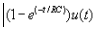Let us call this output of the system S(t). Hence we can say that the response towill be given as follows: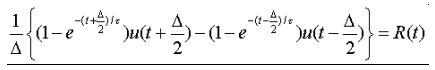Now as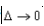the response ofwill  be equal to h(t)

Taking limit as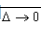on both sides and using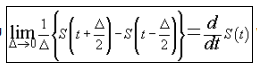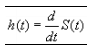By the sifting property we get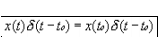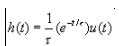Hence if we are given the unit step responseu (t) we have been able to calculate the continuous impulse response of the system. Next we shall see how we can get the unit step response from the impulse response of the same system.

Impulse response of RC system

We have seen how we could calculate the impulse response from the unit step response of a system. Now we shall calculate the unit step response, or in general the response to any input signal, given its impulse response. We shall use convolution to obtain the required result

The unit impulse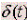, when fed into the RC system gives the corresponding impulse response h(t), which in this case is given by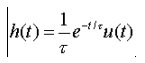We shall now find the response to the input signal u(t).

The convolution of an input signal x(t) and the impulse response of a system h(t) is given by the formula: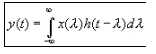But in this case x(t) = u(t), and so the output signal y(t) will be given by :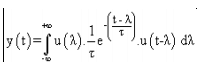Now we have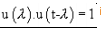if and only if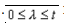In all other cases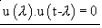Hence the given equation for y(t) will now simplify to :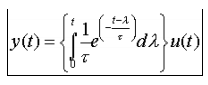Solving which we get ,Solving which we get ,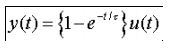which is the response to the unit step function.

Hence we have now shown how to calculate the impulse response given the unit step response and also any response given the impulse response. Moreover we can now say that given either the unit step response or the impulse response we can calculate the response to any other input signals.

Convolution Operation

We now interpret the convolution (x*h)(t) as the common (shaded) area enclosed under the curves x(v) and h(t-v) as v varies over the entire real axis.

x(v) is the given input function, with the independent variable now called v. h(t-v) is the impulse response obtained by inverting h(v) and then shifting it by t units on the v-axis.

As t increases clearly h(t-v) can be considered to be a train moving towards the right, and at each point on the v-axis, the area under the product x(v) and h(t-v) is the value of y(t) at that t.

Conclusion:

In this lecture you have learnt:

• The given input x(t) is approximated with narrow rectangular pulses, each scaled to the appropriate value of x(t) at the corresponding t (which lies at the midpoint of the base of width d). This is called the staircase approximation of x(t).
• By merely knowing the impulse response one can predict the response of the signal x(t) by using the given formula for convolution.
• If we are given unit-step response, we can calculate unit-impulse response by differentiating the unit-step response .
• If we are given unit-impulse response, we can calculate unit-step response by taking running integral of unit-impulse response .
• The convolution (x*h)(t) is the common (shaded) area enclosed under the curves x(v) and h(t-v)as varies over the entire real axis.
• As t increases, h(t-v) can be considered to be a train moving towards the right and at each point on the v -axis the common area under the product x(v) and h(t-v) is the value of y(t) at that t.
The document Continuous Time LTI Systems - Notes | Study Signals and Systems - Electrical Engineering (EE) is a part of the Electrical Engineering (EE) Course Signals and Systems.
All you need of Electrical Engineering (EE) at this link: Electrical Engineering (EE)

## Signals and Systems

40 videos|44 docs|33 tests

## Signals and Systems

40 videos|44 docs|33 tests

Track your progress, build streaks, highlight & save important lessons and more!

,

,

,

,

,

,

,

,

,

,

,

,

,

,

,

,

,

,

,

,

,

;